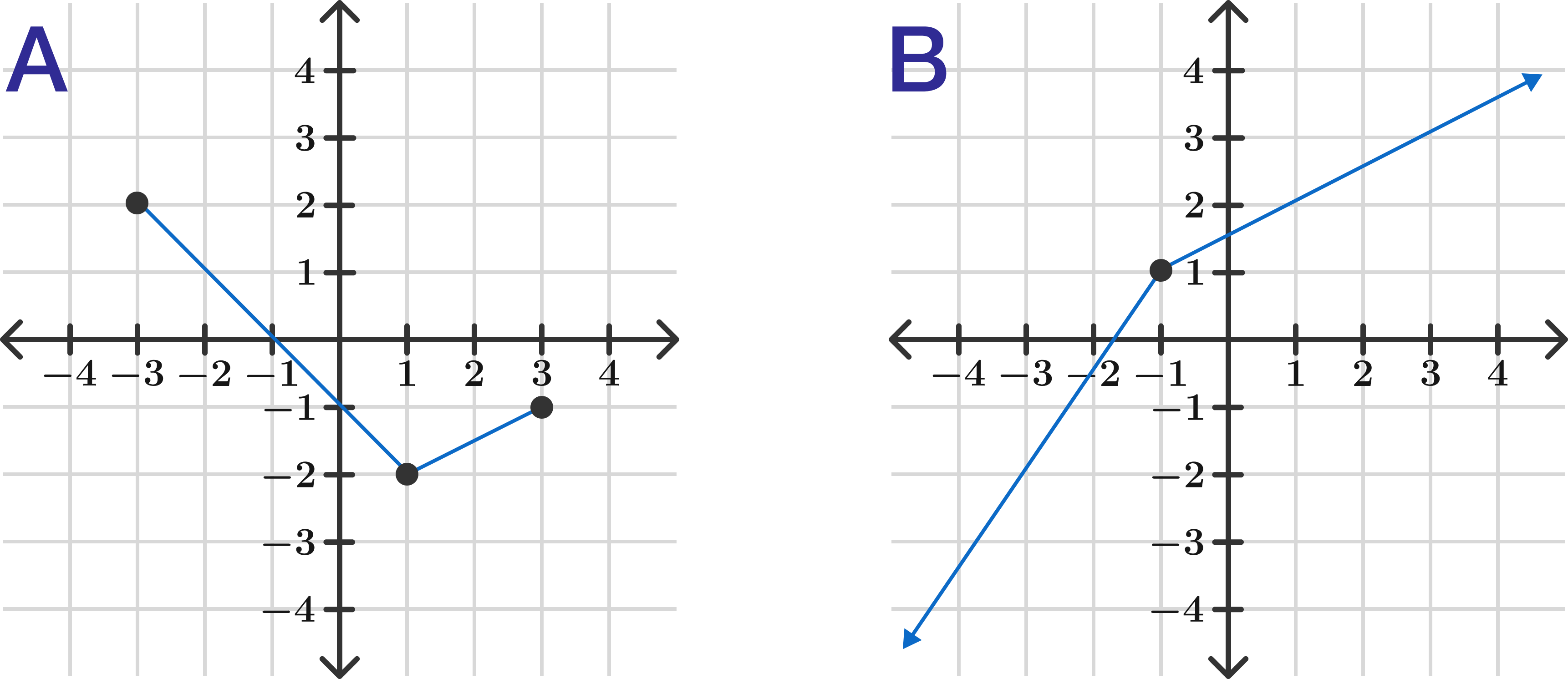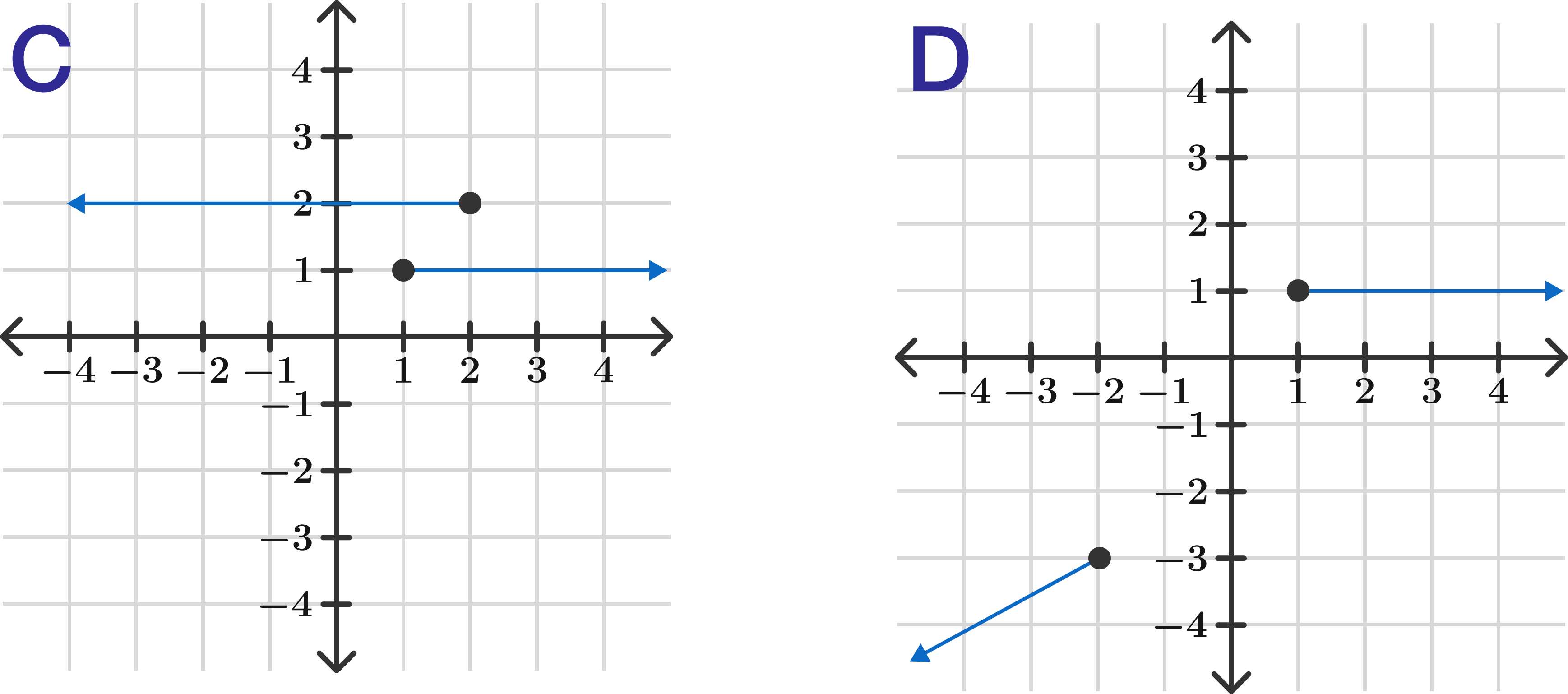# Algebra II: Modeling and Functions

Functions are processes that take some input and produce some output, where every valid input produces only one specific output.

If inputs are on the horizontal $x$-axis and outputs are on the vertical $y$-axis on the graphs below, how many of them are functions?×